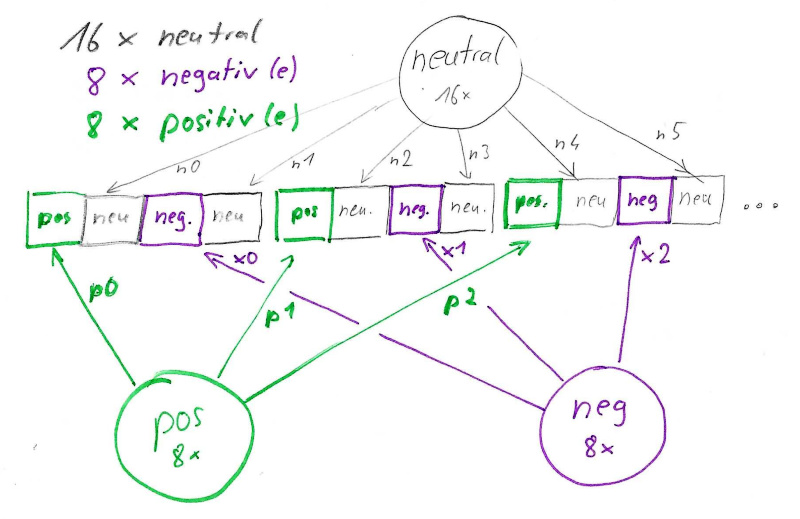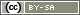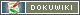# Complex Rotations

This topic is about designs in which rotations should follow specific rules.

In these cases there is no way to avoid a little programming, in which the rules are cast into program code. And yes, admittedly, it's not always trivial. But here are some tips to get you started.

## Basics

### isset()

First of all, you should be familiar with the combination of `isset()` and `registerVariable()`. The PHP function `isset()` checks whether a PHP variable has already been defined. Since PHP variables are normally only valid within a PHP code block, this only becomes interesting in conjunction with `registerVariable()`. This function in fact ensures that the PHP variable is available in all future PHP blocks on all pages.

So we check with `isset()` if a variable – our rotation sequence – already exists. And if not, then we define it (the actual work) and hold it forward using `registerVariable()` so that it is present the next time the page is repeated.

Here once the basic structure. The exclamation mark (`!`) is a negation, so `!isset()` checks if a variable hasn't been defined yet.

```if (!isset(\$sequence)) {
// Here comes the real work,
// to define the desired rotation
\$abfolge = [];
// ...
// ...
// And finally you make the variable available for further
// PHP runs or for further pages.
registerVariable(\$sequence);
}```

Why repeat page? Because the stimuli should usually be presented on separate pages, and for this `loopPage()` is often used, which displays the page over and over again (see below).

### Arrays and shuffle()

For the organization of the sequence, one usually needs one or more lists. Lists are called arrays in PHP. Please read the following chapter so that you know what square brackets in different variations are all about: Introduction to Arrays

The function `shuffle()` then takes over the actual rotation, i.e. the random shuffling of the list. It expects an array as parameter. This is then simply mixed.

Here again the basic framework of defining and shuffling an array.

```\$stimuli = [1,2,3,4,5,6,7,8,9,10];
shuffle(\$stimuli);
// The list could now contain e.g.
// [2,9,3,4,10,7,8,1,6,5]```

Depending on what you are planning for the evaluation, it may be useful to store this sequence in the data set as well. For this the function `putList()` is used. It stores an array in the appropriate number of internal variables.

`putList('IV01', \$stimuli);`

### loopPage()

The function `loopPage()` repeats a page as many times as you like. And, of course, you usually want to display something different each time you run the program. What it is depends on your stimulus.

Let's assume you want to present different images and ask a question about each. Then define the image names as another array and create a corresponding number of questions in the question catalog (“FR01” to “FR10”). And then show one of the pictures and one of the questions in each run. The function `id()` simplifies the creation of the question identifier

```// define list of images
\$images = [
1 => 'stimulus01P.jpg',
2 => 'stimulus01N.jpg',
3 => 'stimulus02P.jpg',
// ...
10 => 'stimulus05N.jpg'
];

// Use a different entry from \$stimuli in each pass
\$imageID = loopPage(\$stimuli);

// Bild anzeigen
\$filename = \$images[\$imageID];
html('
<div style="text-align: center; margin: 2em 0">
<img src="" alt="" style="max-width: 100%">
</div>
');

// Show questions
\$questionID = id('FR', \$imageID);
question(\$questionID);```

Warning: `'loopPage()` is not compatible with `setPageOrder()`, `loopToPage()` or `setNextPage()`. Do not interlace these functions, e.g. do not use `loopPage()` in a page order previously defined by `setPageorder()`.

### FOR-Loops

In programming, the term “loop” means that the same code is repeated several times. Kind of like `loopPage()`, except it's done on the same page in the same PHP code. And just like `loopPage()`, this only becomes interesting if you change something on each iteration.

One possibility is the FOR loop, where a number is incremented and defined as a variable. The following FOR loop counts through the variable `\$i` from 0 to 9. So 10 repetition. Why start with 0? Because it is often easier to calculate and the array indices in PHP (as in many other programming languages) start at 0.

```for (\$i=0; \$i<10; \$i++) {
// ...
}```

The `\$i++` says that `\$i` is incremented by one in each pass. Between the curly brackets (`{…}`)) is then what should happen in each pass.

Only as an example: We could use such a loop to copy the array `\$stimuli` element-by-element into a new array `\$sequence`. This is not efficient, but demonstrates the basic idea.

```\$sequence = [];
for (\$i=0; \$i<10; \$i++) {
\$sequence[] = \$stimuli[\$i];
}```

As you read in the Introduction to Arrays, `\$sequence[] = …` writes a new element to the end of the array `\$sequence`.

### Logic

With these building blocks, complex rotations can now be built and presented in the questionnaire.

But now the challenging part comes: The scheme must be put on paper and then on the display. Putting it on paper means actually sketching out once what comes from where and needs to go where.

Let's take the following example: There is a total of 32 images for the study, these are assigned to 3 categories:

• 16 images are neutral,
• 8 images are positive and
• 8 images are negativ.

Now a positive picture is to be presented in the questionnaire, then a neutral one, then a negative one and then again a neutral one, and this until all pictures are through. And apart from that, of course, the sequence is to be rotated. Sketched on a piece of paper, it might look like this:So we need 3 urns (yes, instead of `shuffle()` you could also use a random generator), from which we draw the images. In the sketch they are circles. In the programming, of course, these are the arrays. The array index of the image is written on the arrows.

In first place is a positive image, the first from the positive list, so it has index 0. Then comes a neutral image. This is also the first from the neutral list, so it also has index 0. Then a negative one and then it gets interesting: The last image in the first block is neutral again. Therefore we have to use another one, so we need the index 1.

In the second block, the indices are then positive:1, neutral:2, negative:1 and neutral:3. The first example below shows how this is then implemented in the programming.

It is still important to know that we repeat this block of 4 images 8 times to present all 32 images.

## Repeat Scheme

The first example has already been explained above in the section logic: We would like to present 32 images (neutral, positive, negative) according to a fixed scheme – but rotated.

We also already know that we need 3 arrays and have to assemble 8 blocks of 4 images each from them.

### Step 1

In the first step we abstract from the file names and assign numbers to the images. For clarity, they are numbers 1 through 16 for the neutral images, 21 through 28 for the positive images, and 31 through 38 for the negative images.

Why do we do this? Because then we only have to shuffle numbers. This has two advantages:

• Numbers can be saved more easily in the data set and
• We can create questions matching the numbers, which are then fixed to an image. The question “BE22” would be fix connected with the second positive image.
```\$images = [
// neutral images
1 => 'image.N1.jpg',
2 => 'image.N2.jpg',
3 => 'image.N3.jpg',
// ...
16 => 'image.N16.jpg',

// positive images
21 => 'image.P1.jpg',
// ...
28 => 'image.P8.jpg',

// negative images
31 => 'image.N1.jpg',
// ...
38 => 'image.N8.jpg'
];```

The dots (`…`) have to be filled with image names of course.

### Step 2

In the second step we define the three lists and shuffle them using `shuffle()`. Here we only put the numbers of the images into the arrays.

```\$neutral = [1,2,3, ... 16];
\$positive = [21,22,23, ... 28];
\$negative = [31,32,33, ... 38];
shuffle(\$neutral);
shuffle(\$positive);
shuffle(\$negative);```

### Step 3

Then the total list of 32 image codes, consisting of 8 blocks of 4 images each, is assembled. Here a FOR loop is used. One pass for each (similar) block.

Above we had explained that in the first run we need the following indices (counting starts at 0) from the three lists:

• positive:0, neutral:0, negative:0 and neutral:1

In the second and third pass we then need the following indices (see sketch above):

• positive:1, neutral:2, negative:1 and neutral:3
• positive:2, neutral:4, negative:2 and neutral:5

How do we get from a count variable `\$i` of the FOR loop `[0,1,2,…,7]` to these values? For the positive and negative entries it is trivial, there we can use the variable directly. For the neutral entries we have to calculate. Spoiler: We don't have to count from 1 to 8 at the end but from 1 to 16, so we multiply by 2. And for the second value in the block, we simply add 1.

• for `\$i=0` we get 0 (2-mal 0) and 1
• for `\$i=1` we get 2 (2-mal 1) and 3
• for `\$i=2` we get 4 (2-mal 2) and 5
• and so on, so exactly what we need

What about the FOR loop and counting and assembling in PHP code?

```// First we need an empty list, where the new entries will go in
\$sequence = [];
for (\$i=0; \$i<8; \$i++) {
\$sequence[] = \$positive[\$i];
\$sequence[] = \$neutral[2 * \$i];
\$sequence[] = \$negative[\$i];
\$sequence[] = \$neutral[2 * \$i + 1];
}```

So in each pass 4 values are added to the array `\$flow`. And always the ones we wanted to have according to the sketch above.

### Step 4

Finally, we would like to display the images and associated questions (“BE01” to “BE38”) in the questionnaire, of course. And on individual pages. For this now `loopPage()` is used. It uses the previously defined list and works through it entry by entry.

```\$imageID = loopPage(\$sequence);

// show image
\$filename = \$image[\$imageID];
html('
<div style="text-align: center; margin: 2em 0">
<img src="" alt="" style="max-width: 100%">
</div>
');

// show question
\$questionID = id('BE', \$imageID);
question(\$questionID);```

Of course, you could also store page names in the sequence and pass them to `setPageOrder()` if you want to present pages in a certain order. But with `loopPage()` you have to create only one page instead of 32 pages. This is much faster, clearer and less error-prone.

The HTML code to display the images is explained here: Images in the Questionnaire und Single Factorial Design

### Assemble Everything

The last step is to assemble everything. And we need `isset()` and `registerVariable()` so that the sequence is not shuffled again with each repetition.

We also create an internal variable `IV01` with 32 variables to store the sequence as well. In case we want to control for series effects in the evaluation. The image list (`\$images`) is better redefined each time, then we don't need to store the image names in each interview, that would just waste memory.

```\$images = [
// neutral images
1 => 'image.N1.jpg',
2 => 'image.N2.jpg',
3 => 'image.N3.jpg',
// ...
16 => 'image.N16.jpg',
// positive images
21 => 'image.P1.jpg',
// ...
28 => 'image.P8.jpg',
// negative images
31 => 'image.N1.jpg',
// ...
38 => 'image.N8.jpg'
];

if (!isset(\$sequence)) {
\$neutral = [1,2,3, ... 16];
\$positive = [21,22,23, ... 28];
\$negative = [31,32,33, ... 38];
shuffle(\$neutral);
shuffle(\$positive);
shuffle(\$negative);

\$sequence = [];
for (\$i=0; \$i<8; \$i++) {
\$sequence[] = \$positive[\$i];
\$sequence[] = \$neutral[2 * \$i];
\$sequence[] = \$negative[\$i];
\$sequence[] = \$neutral[2 * \$i + 1];
}

// Store sequence also in data set
putList('IV01', \$sequence);

registerVariable(\$sequence);
}

// Present entries each on a separate page
\$imageID = loopPage(\$sequence);

// show image
\$filename = \$images[\$imageID];
html('
<div style="text-align: center; margin: 2em 0">
<img src="" alt="" style="max-width: 100%">
</div>
');

// show question
\$questionID = id('BE', \$imageID);
question(\$questionID);```